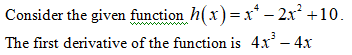# 10. At what points (if any) (x, y) does the following function have a horizontal tangent line?Does it have a vertical tangent line? Show work to justify your answers.h(x) = x^4-2x^2+10

Question
1 views

10. At what points (if any) (x, y) does the following function have a horizontal tangent line?
Does it have a vertical tangent line? Show work to justify your answers.
h(x) = x^4-2x^2+10

check_circle

Step 1Step 2

Now, equate the first derivative to zero and find the values of x. Finally plug in the values of x in the original equation.

Step 3

Now, substitute the values of x in ...

### Want to see the full answer?

See Solution

#### Want to see this answer and more?

Solutions are written by subject experts who are available 24/7. Questions are typically answered within 1 hour.*

See Solution
*Response times may vary by subject and question.
Tagged in

### Functions